# GMAT Math : DSQ: Calculating the perimeter of a polygon

## Example Questions

### Example Question #1 : Polygons

Given a regular hexagon and a regular octagon, which, if either, has the greater perimeter?

Statement 1: The sidelength of the octagon is one foot.

Statement 2: The sidelength of the hexagon is fifteen inches.

BOTH statements TOGETHER are sufficient to answer the question, but NEITHER statement ALONE is sufficient to answer the question.

Statement 1 ALONE is sufficient to answer the question, but Statement 2 ALONE is NOT sufficient to answer the question.

EITHER statement ALONE is sufficient to answer the question.

Statement 2 ALONE is sufficient to answer the question, but Statement 1 ALONE is NOT sufficient to answer the question.

BOTH statements TOGETHER are insufficient to answer the question.

BOTH statements TOGETHER are sufficient to answer the question, but NEITHER statement ALONE is sufficient to answer the question.

Explanation:

Each of the two statements allows you to find out the perimeter of one of the polygons by multiplying its sidelength by the number of its sides. However, neither statement offers any clues to the perimeter of the other polygon. Both statements together, however, allow you to determine and to compare both perimeters.

### Example Question #2 : Polygons

You are given a regular hexagon and a regular pentagon. Which one has the greater perimeter?

Statement 1: The hexagon and the pentagon have the same area

Statement 2: The apothem of the hexagon is greater than that of the pentagon

Statement 2 ALONE is sufficient to answer the question, but Statement 1 ALONE is NOT sufficient to answer the question.

Statement 1 ALONE is sufficient to answer the question, but Statement 2 ALONE is NOT sufficient to answer the question.

EITHER statement ALONE is sufficient to answer the question.

BOTH statements TOGETHER are insufficient to answer the question.

BOTH statements TOGETHER are sufficient to answer the question, but NEITHER statement ALONE is sufficient to answer the question.

BOTH statements TOGETHER are sufficient to answer the question, but NEITHER statement ALONE is sufficient to answer the question.

Explanation:

The area of a polygon is one-half the product of its perimeter and its apothem (the perpendicular distance from the center to a side). Therefore,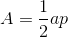or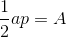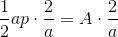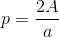Therefore, the perimeter can be determined from both area and apothem, but not from one alone. Neither statement alone gives you enough information, but from both statements together, it can be determined that the pentagon has the greater perimeter.

### Example Question #3 : Polygons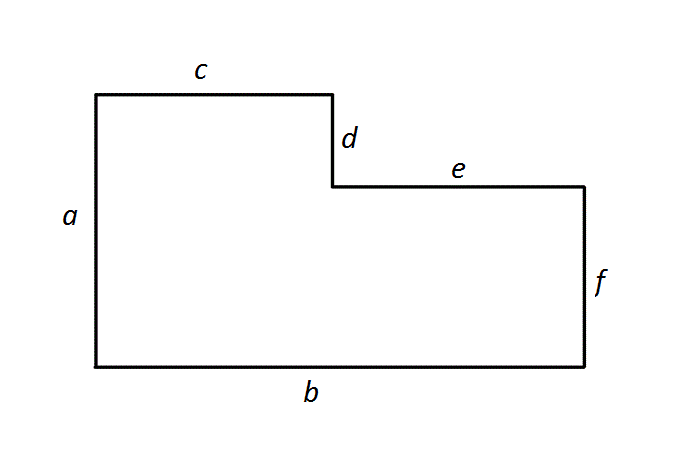Note: Figure NOT drawn to scale. All angles shown are right angles.

What is the perimeter of the above figure?

Statement 1: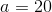Statement 2: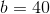Statement 1 ALONE is sufficient to answer the question, but Statement 2 ALONE is NOT sufficient to answer the question.

Statement 2 ALONE is sufficient to answer the question, but Statement 1 ALONE is NOT sufficient to answer the question.

EITHER statement ALONE is sufficient to answer the question.

BOTH statements TOGETHER are sufficient to answer the question, but NEITHER statement ALONE is sufficient to answer the question.

BOTH statements TOGETHER are insufficient to answer the question.

BOTH statements TOGETHER are sufficient to answer the question, but NEITHER statement ALONE is sufficient to answer the question.

Explanation:

The figure can be seen as a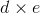rectangle cut out of an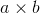rectangle.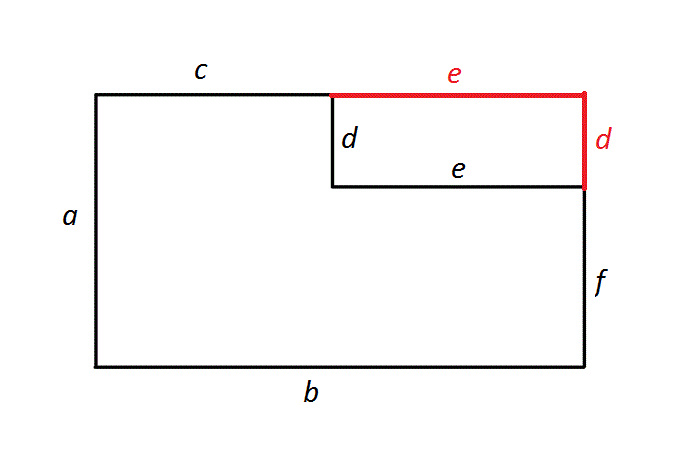The perimeter of the composite figure is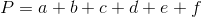.

However, since opposite sides of a rectangle are congruent, then, as can be seen in the figure,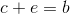and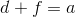.

The perimeter can then be rewritten: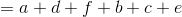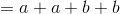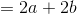Therefore, it is necessary and sufficient to knowand; the other four sidelengths are not needed to determine the perimeter of the figure.

### Example Question #4 : Polygons

Consider regular decagon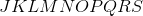.

I) Side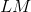is 56 inches long.

II) Side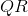plus Side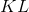is equivalent to 112 inches.

Find the perimeter of.

Statement II is sufficient to answer the question, but Statement I is not sufficient to answer the question.

Both statements are needed to answer the question.

Statement I is sufficient to answer the question, but Statement II is not sufficient to answer the question.

Either statement is sufficient to answer the question.

Either statement is sufficient to answer the question.

Explanation:

To find perimeter, we need the length of all the sides. Note that we are dealing with a regular polygon, so all of its sides are equal.

Statement I gives us one side length. Multiply the side by ten (decagons have ten sides):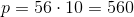Statement II gives us the length of two sides together. Multiply by 5 to get the total perimeter: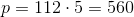### Example Question #5 : Polygons

Consider pentagon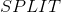.

I) Side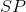has the same length as side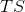, 5 inches.

II) Sideis one-third the length of side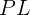, and sides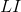and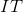have the same length as side.

What is the perimeter ofBoth statements are needed to answer the question.

Statement II is sufficient to answer the question, but Statement I is not sufficient to answer the question.

Statement I is sufficient to answer the question, but Statement II is not sufficient to answer the question.

Either statement is sufficient to answer the question.

Both statements are needed to answer the question.

Explanation:

Consider pentagon.

I) Sidehas the same length as side, 5 inches.

II) Sideis one-third the length of sidesand.

What is the perimeter ofTo find perimeter, we need to know the length of all the sides.

Statement I gives us the lengths of two sides.

Statement II relates one of the sides given in Statement I to the other three sides: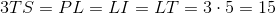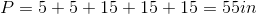Tired of practice problems?

Try live online GMAT prep today.